Скачать презентацию Chapter 5 Part 2 Mass and Energy Analysis

35b786211a9d82cfa6083f1750df7421.ppt

• Количество слайдов: 43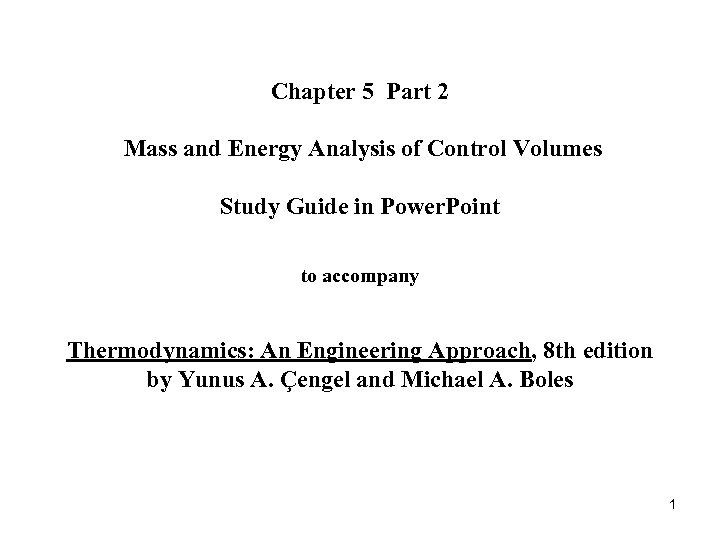Chapter 5 Part 2 Mass and Energy Analysis of Control Volumes Study Guide in Power. Point to accompany Thermodynamics: An Engineering Approach, 8 th edition by Yunus A. Çengel and Michael A. Boles 1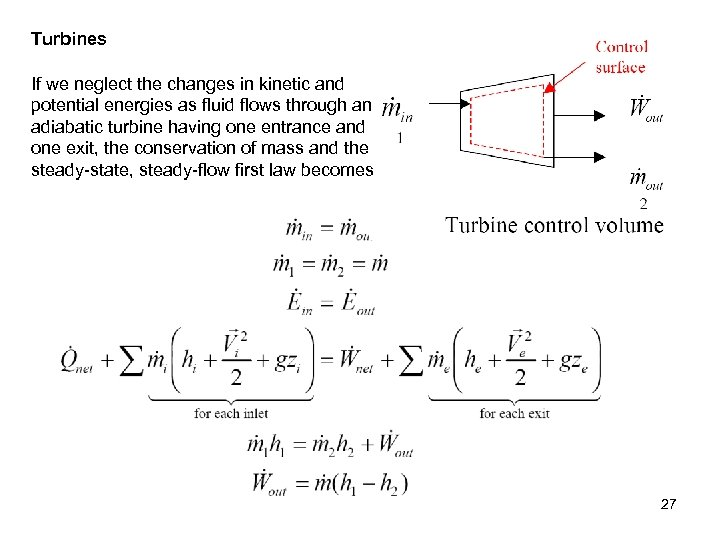Turbines If we neglect the changes in kinetic and potential energies as fluid flows through an adiabatic turbine having one entrance and one exit, the conservation of mass and the steady-state, steady-flow first law becomes 27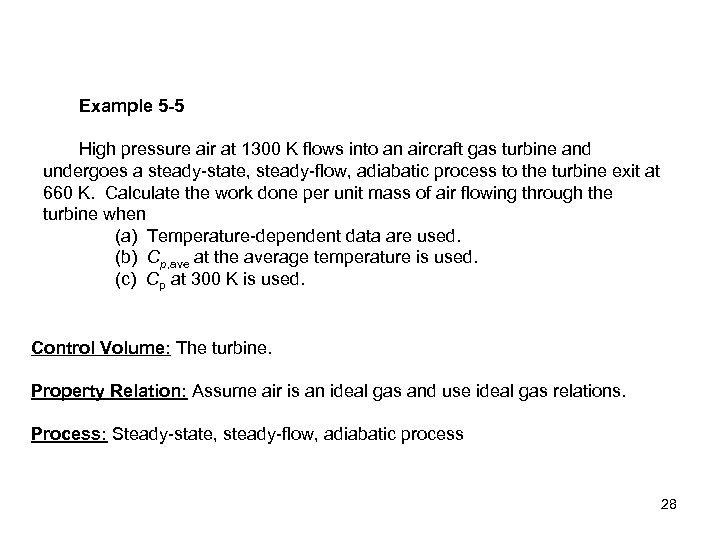Example 5 -5 High pressure air at 1300 K flows into an aircraft gas turbine and undergoes a steady-state, steady-flow, adiabatic process to the turbine exit at 660 K. Calculate the work done per unit mass of air flowing through the turbine when (a) Temperature-dependent data are used. (b) Cp, ave at the average temperature is used. (c) Cp at 300 K is used. Control Volume: The turbine. Property Relation: Assume air is an ideal gas and use ideal gas relations. Process: Steady-state, steady-flow, adiabatic process 28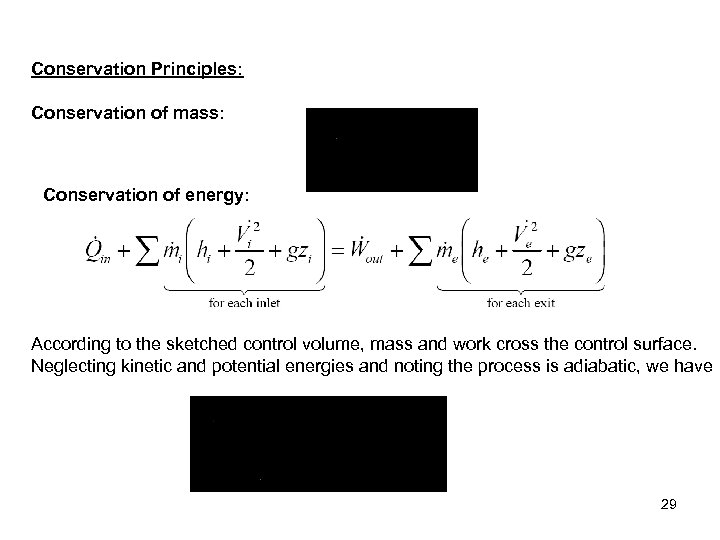Conservation Principles: Conservation of mass: Conservation of energy: According to the sketched control volume, mass and work cross the control surface. Neglecting kinetic and potential energies and noting the process is adiabatic, we have 29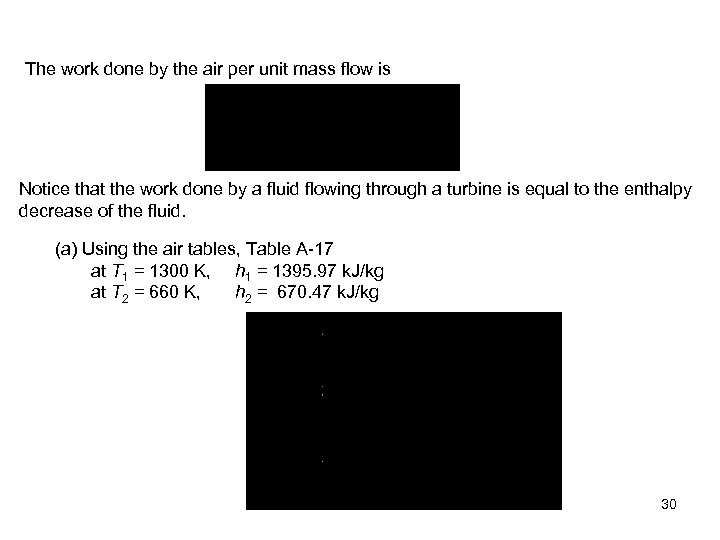The work done by the air per unit mass flow is Notice that the work done by a fluid flowing through a turbine is equal to the enthalpy decrease of the fluid. (a) Using the air tables, Table A-17 at T 1 = 1300 K, h 1 = 1395. 97 k. J/kg at T 2 = 660 K, h 2 = 670. 47 k. J/kg 30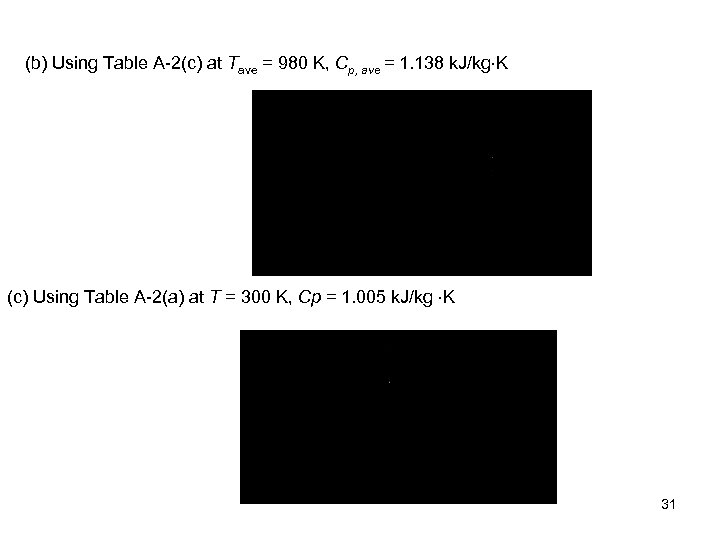(b) Using Table A-2(c) at Tave = 980 K, Cp, ave = 1. 138 k. J/kg K (c) Using Table A-2(a) at T = 300 K, Cp = 1. 005 k. J/kg K 31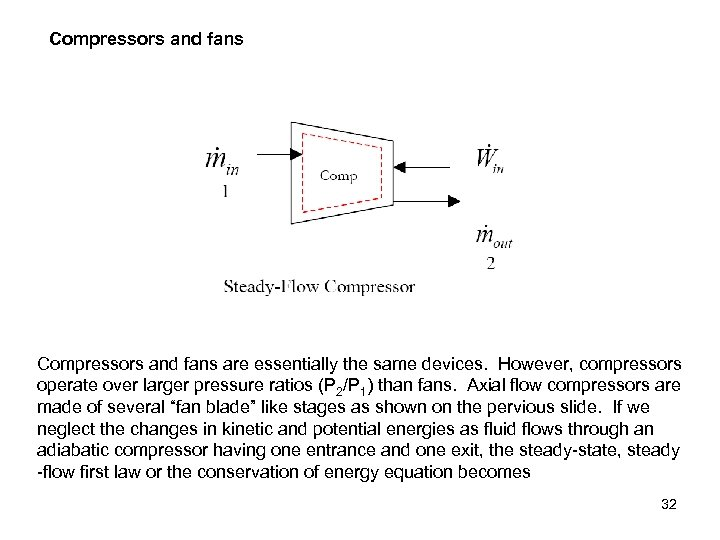Compressors and fans are essentially the same devices. However, compressors operate over larger pressure ratios (P 2/P 1) than fans. Axial flow compressors are made of several “fan blade” like stages as shown on the pervious slide. If we neglect the changes in kinetic and potential energies as fluid flows through an adiabatic compressor having one entrance and one exit, the steady-state, steady -flow first law or the conservation of energy equation becomes 32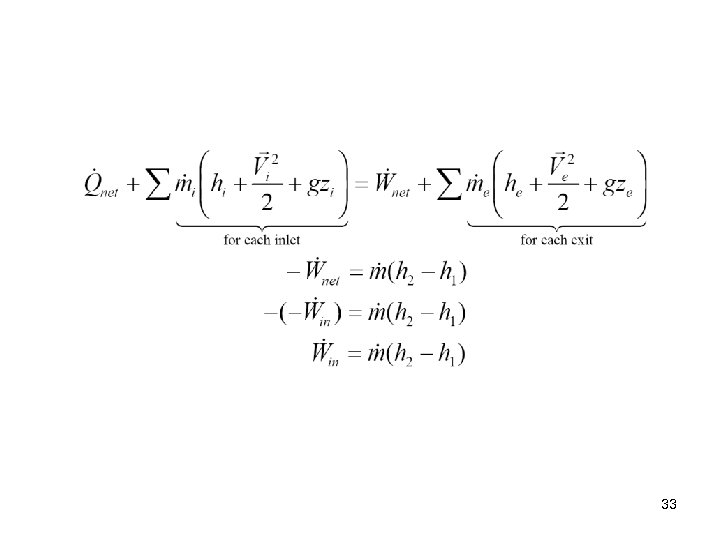33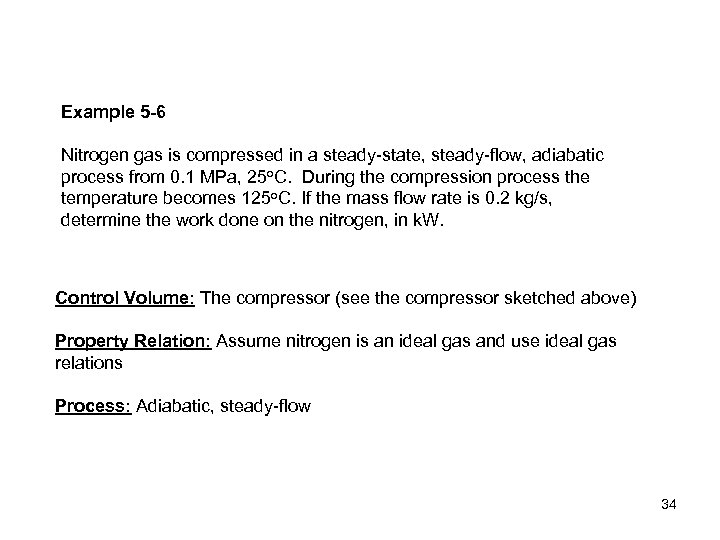Example 5 -6 Nitrogen gas is compressed in a steady-state, steady-flow, adiabatic process from 0. 1 MPa, 25 o. C. During the compression process the temperature becomes 125 o. C. If the mass flow rate is 0. 2 kg/s, determine the work done on the nitrogen, in k. W. Control Volume: The compressor (see the compressor sketched above) Property Relation: Assume nitrogen is an ideal gas and use ideal gas relations Process: Adiabatic, steady-flow 34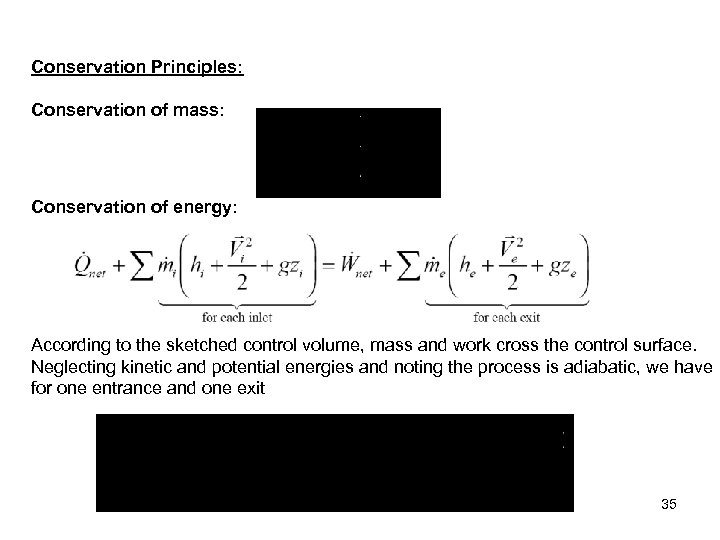Conservation Principles: Conservation of mass: Conservation of energy: According to the sketched control volume, mass and work cross the control surface. Neglecting kinetic and potential energies and noting the process is adiabatic, we have for one entrance and one exit 35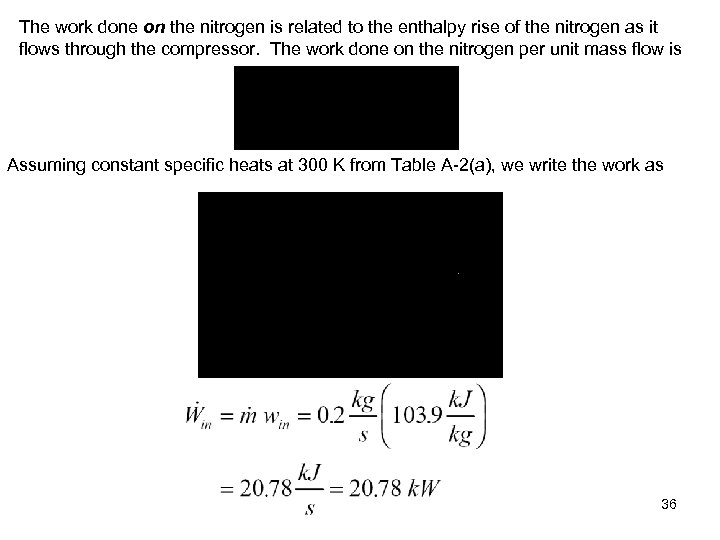The work done on the nitrogen is related to the enthalpy rise of the nitrogen as it flows through the compressor. The work done on the nitrogen per unit mass flow is Assuming constant specific heats at 300 K from Table A-2(a), we write the work as 36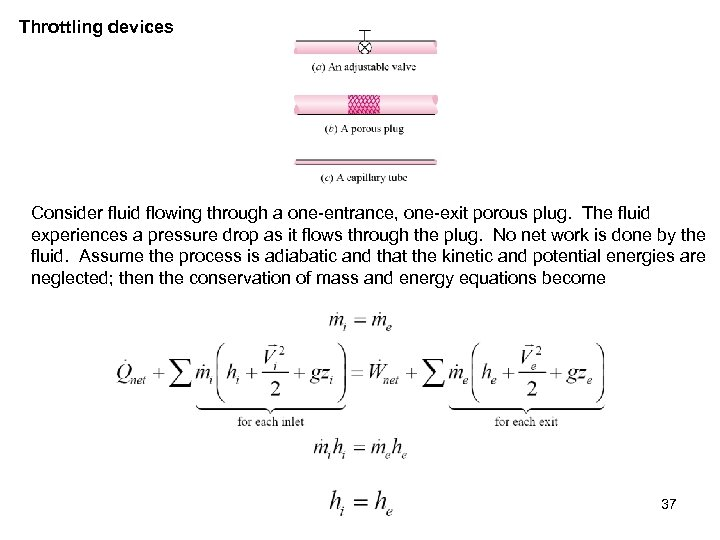Throttling devices Consider fluid flowing through a one-entrance, one-exit porous plug. The fluid experiences a pressure drop as it flows through the plug. No net work is done by the fluid. Assume the process is adiabatic and that the kinetic and potential energies are neglected; then the conservation of mass and energy equations become 37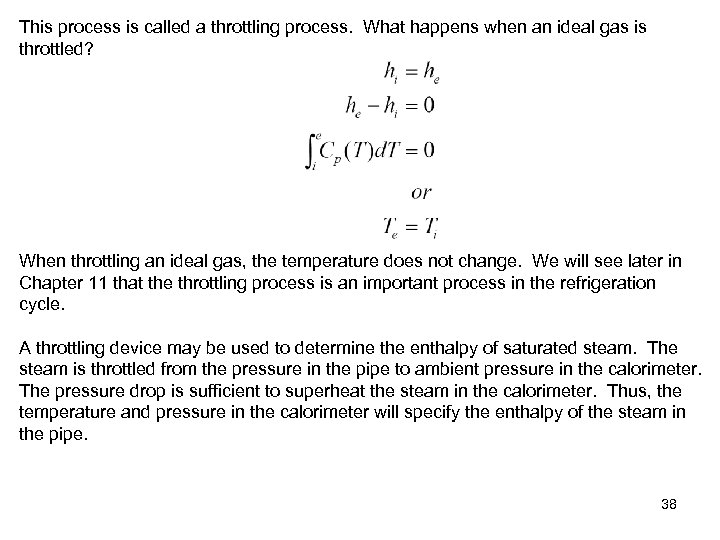This process is called a throttling process. What happens when an ideal gas is throttled? When throttling an ideal gas, the temperature does not change. We will see later in Chapter 11 that the throttling process is an important process in the refrigeration cycle. A throttling device may be used to determine the enthalpy of saturated steam. The steam is throttled from the pressure in the pipe to ambient pressure in the calorimeter. The pressure drop is sufficient to superheat the steam in the calorimeter. Thus, the temperature and pressure in the calorimeter will specify the enthalpy of the steam in the pipe. 38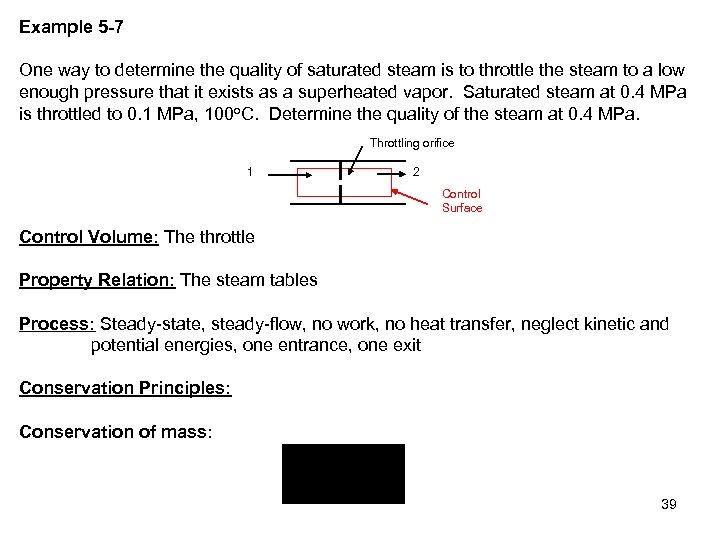Example 5 -7 One way to determine the quality of saturated steam is to throttle the steam to a low enough pressure that it exists as a superheated vapor. Saturated steam at 0. 4 MPa is throttled to 0. 1 MPa, 100 o. C. Determine the quality of the steam at 0. 4 MPa. Throttling orifice 1 2 Control Surface Control Volume: The throttle Property Relation: The steam tables Process: Steady-state, steady-flow, no work, no heat transfer, neglect kinetic and potential energies, one entrance, one exit Conservation Principles: Conservation of mass: 39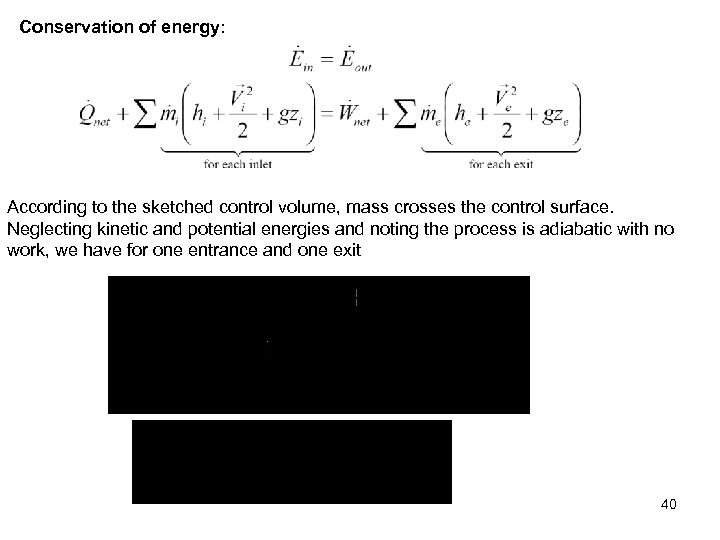Conservation of energy: According to the sketched control volume, mass crosses the control surface. Neglecting kinetic and potential energies and noting the process is adiabatic with no work, we have for one entrance and one exit 40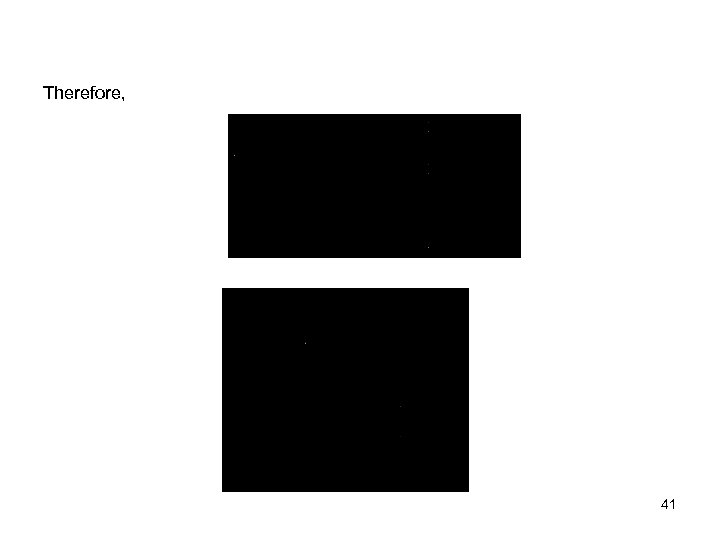Therefore, 41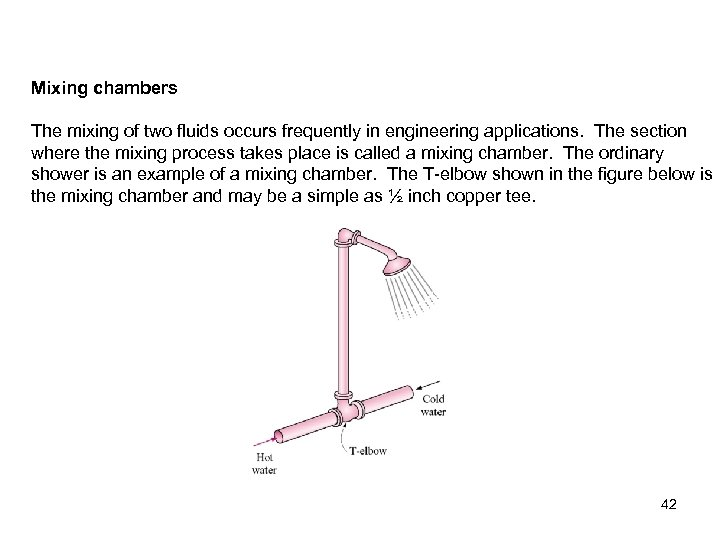Mixing chambers The mixing of two fluids occurs frequently in engineering applications. The section where the mixing process takes place is called a mixing chamber. The ordinary shower is an example of a mixing chamber. The T-elbow shown in the figure below is the mixing chamber and may be a simple as ½ inch copper tee. 42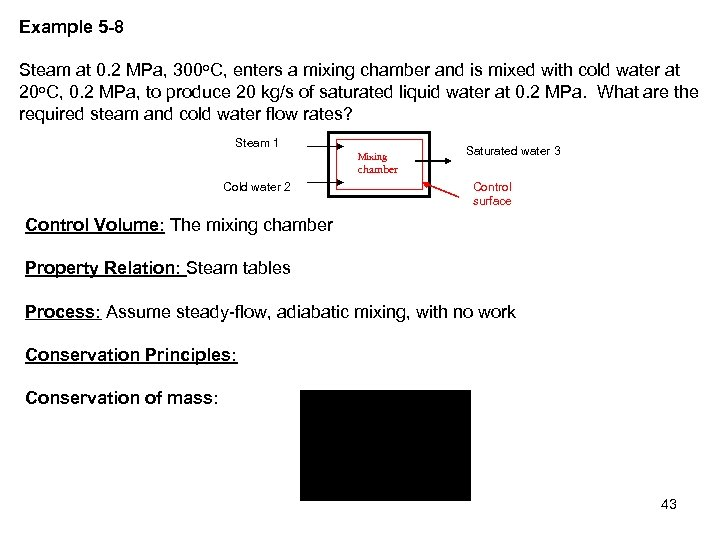Example 5 -8 Steam at 0. 2 MPa, 300 o. C, enters a mixing chamber and is mixed with cold water at 20 o. C, 0. 2 MPa, to produce 20 kg/s of saturated liquid water at 0. 2 MPa. What are the required steam and cold water flow rates? Steam 1 Mixing Saturated water 3 chamber Cold water 2 Control surface Control Volume: The mixing chamber Property Relation: Steam tables Process: Assume steady-flow, adiabatic mixing, with no work Conservation Principles: Conservation of mass: 43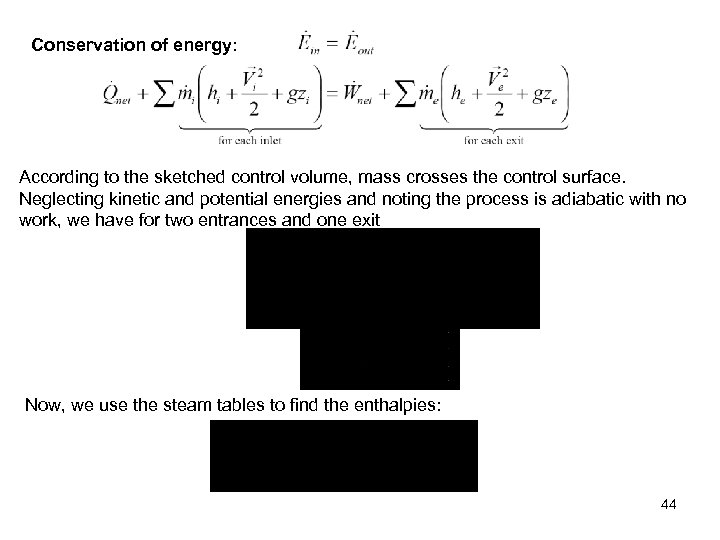Conservation of energy: According to the sketched control volume, mass crosses the control surface. Neglecting kinetic and potential energies and noting the process is adiabatic with no work, we have for two entrances and one exit Now, we use the steam tables to find the enthalpies: 44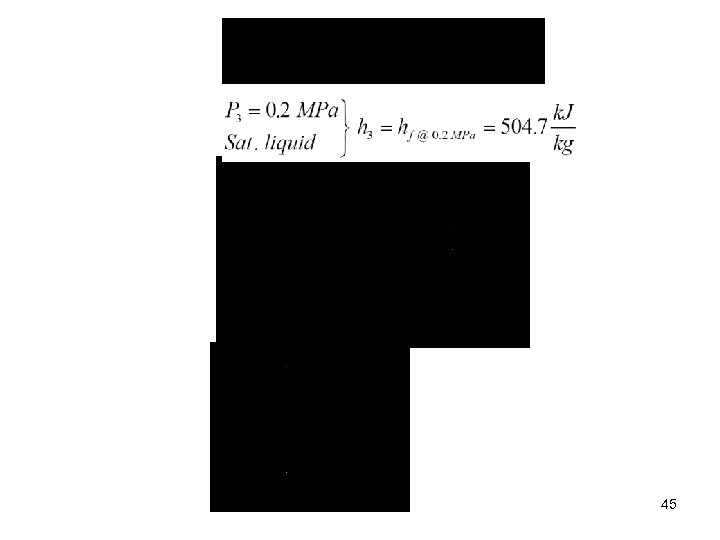45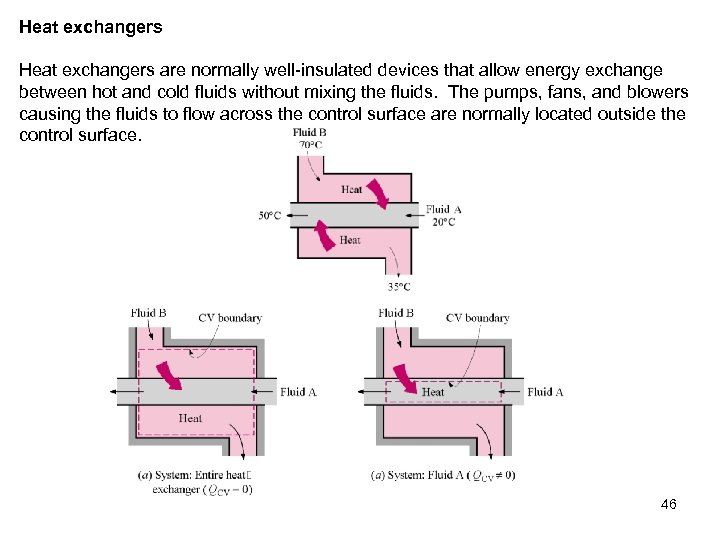Heat exchangers are normally well-insulated devices that allow energy exchange between hot and cold fluids without mixing the fluids. The pumps, fans, and blowers causing the fluids to flow across the control surface are normally located outside the control surface. 46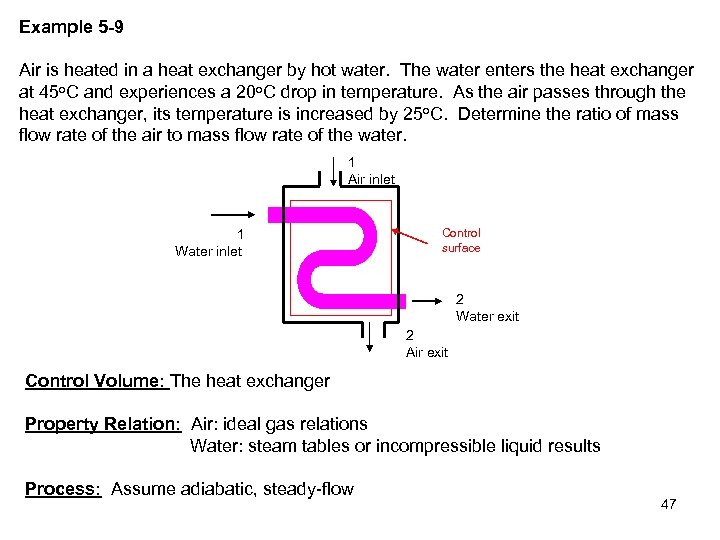Example 5 -9 Air is heated in a heat exchanger by hot water. The water enters the heat exchanger at 45 o. C and experiences a 20 o. C drop in temperature. As the air passes through the heat exchanger, its temperature is increased by 25 o. C. Determine the ratio of mass flow rate of the air to mass flow rate of the water. 1 Air inlet 1 Water inlet Control surface 2 Water exit 2 Air exit Control Volume: The heat exchanger Property Relation: Air: ideal gas relations Water: steam tables or incompressible liquid results Process: Assume adiabatic, steady-flow 47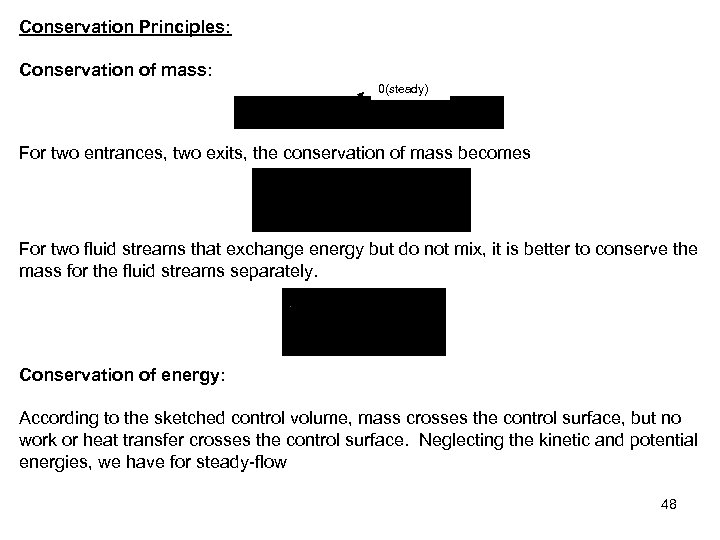Conservation Principles: Conservation of mass: 0(steady) For two entrances, two exits, the conservation of mass becomes For two fluid streams that exchange energy but do not mix, it is better to conserve the mass for the fluid streams separately. Conservation of energy: According to the sketched control volume, mass crosses the control surface, but no work or heat transfer crosses the control surface. Neglecting the kinetic and potential energies, we have for steady-flow 48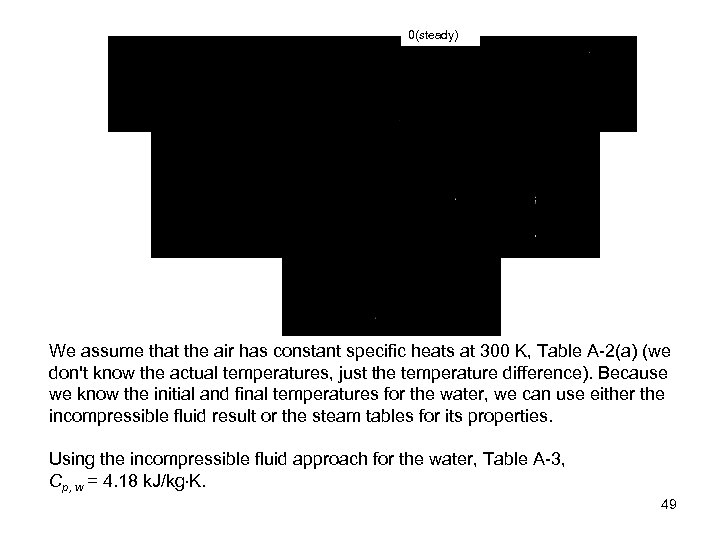0(steady) We assume that the air has constant specific heats at 300 K, Table A-2(a) (we don't know the actual temperatures, just the temperature difference). Because we know the initial and final temperatures for the water, we can use either the incompressible fluid result or the steam tables for its properties. Using the incompressible fluid approach for the water, Table A-3, Cp, w = 4. 18 k. J/kg K. 49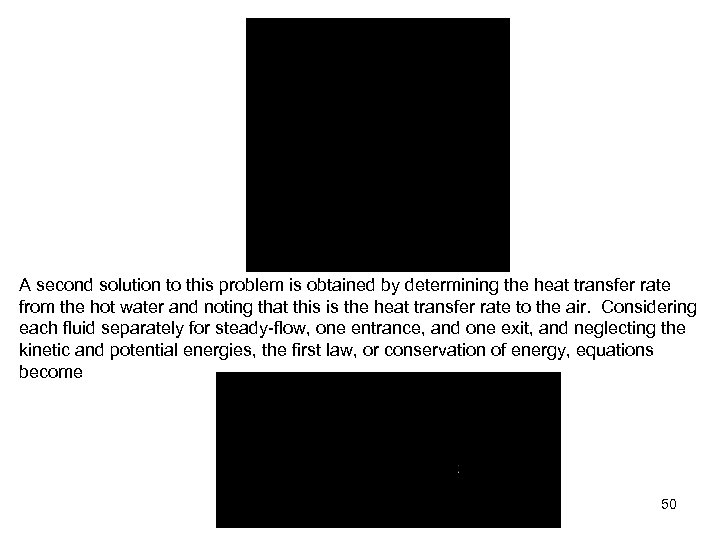A second solution to this problem is obtained by determining the heat transfer rate from the hot water and noting that this is the heat transfer rate to the air. Considering each fluid separately for steady-flow, one entrance, and one exit, and neglecting the kinetic and potential energies, the first law, or conservation of energy, equations become 50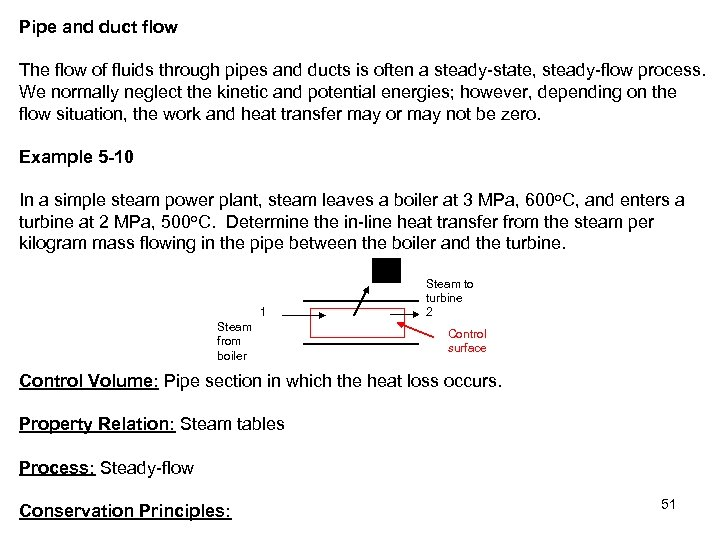Pipe and duct flow The flow of fluids through pipes and ducts is often a steady-state, steady-flow process. We normally neglect the kinetic and potential energies; however, depending on the flow situation, the work and heat transfer may or may not be zero. Example 5 -10 In a simple steam power plant, steam leaves a boiler at 3 MPa, 600 o. C, and enters a turbine at 2 MPa, 500 o. C. Determine the in-line heat transfer from the steam per kilogram mass flowing in the pipe between the boiler and the turbine. 1 Steam from boiler Steam to turbine 2 Control surface Control Volume: Pipe section in which the heat loss occurs. Property Relation: Steam tables Process: Steady-flow Conservation Principles: 51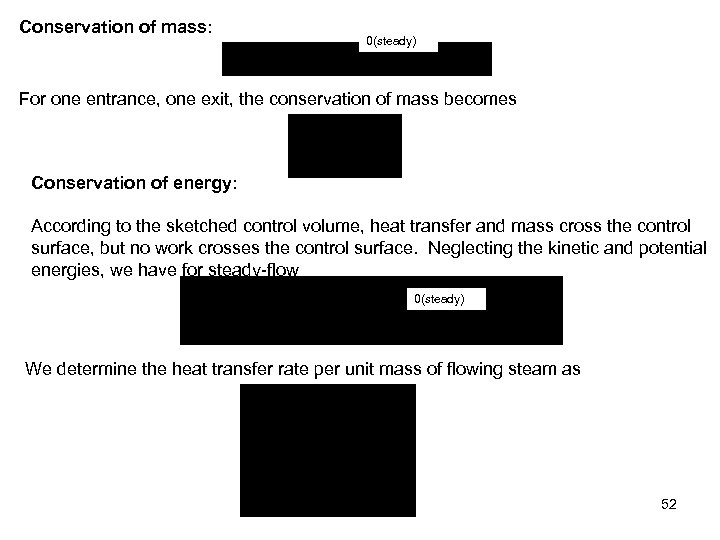Conservation of mass: 0(steady) For one entrance, one exit, the conservation of mass becomes Conservation of energy: According to the sketched control volume, heat transfer and mass cross the control surface, but no work crosses the control surface. Neglecting the kinetic and potential energies, we have for steady-flow 0(steady) We determine the heat transfer rate per unit mass of flowing steam as 52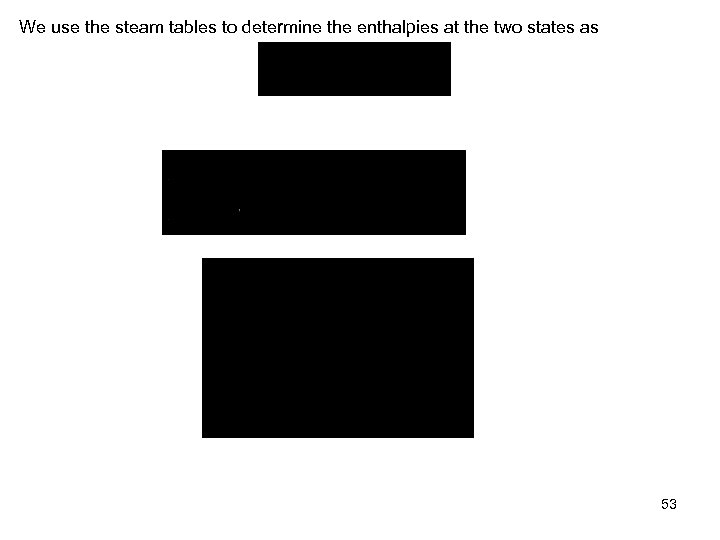We use the steam tables to determine the enthalpies at the two states as 53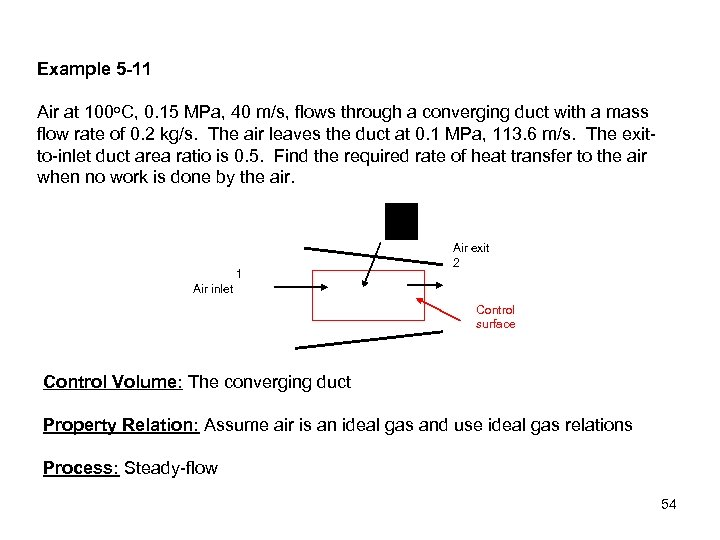Example 5 -11 Air at 100 o. C, 0. 15 MPa, 40 m/s, flows through a converging duct with a mass flow rate of 0. 2 kg/s. The air leaves the duct at 0. 1 MPa, 113. 6 m/s. The exitto-inlet duct area ratio is 0. 5. Find the required rate of heat transfer to the air when no work is done by the air. 1 Air exit 2 Air inlet Control surface Control Volume: The converging duct Property Relation: Assume air is an ideal gas and use ideal gas relations Process: Steady-flow 54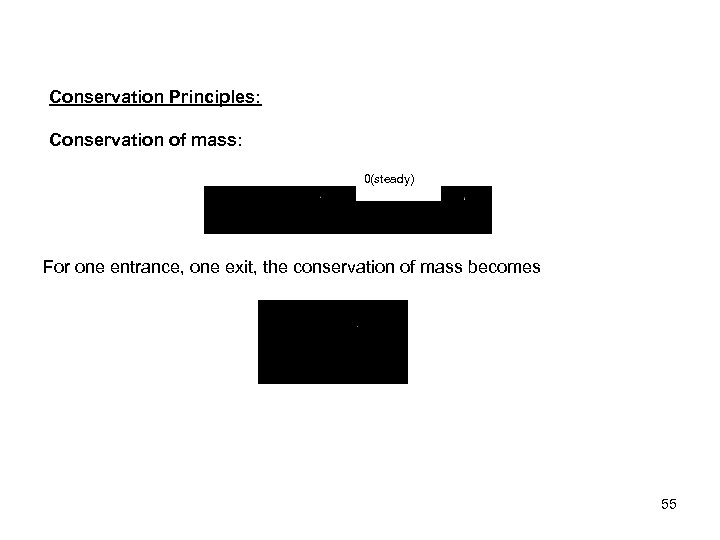Conservation Principles: Conservation of mass: 0(steady) For one entrance, one exit, the conservation of mass becomes 55Conservation of energy: According to the sketched control volume, heat transfer and mass cross the control surface, but no work crosses the control surface. Here keep the kinetic energy and still neglect the potential energies, we have for steady-state, steady-flow process 0(steady) In the first law equation, the following are known: P 1, T 1 (and h 1), , and A 2/A 1. The unknowns are , and h 2 (or T 2). We use the first law and the conservation of mass equation to solve for the two unknowns. 56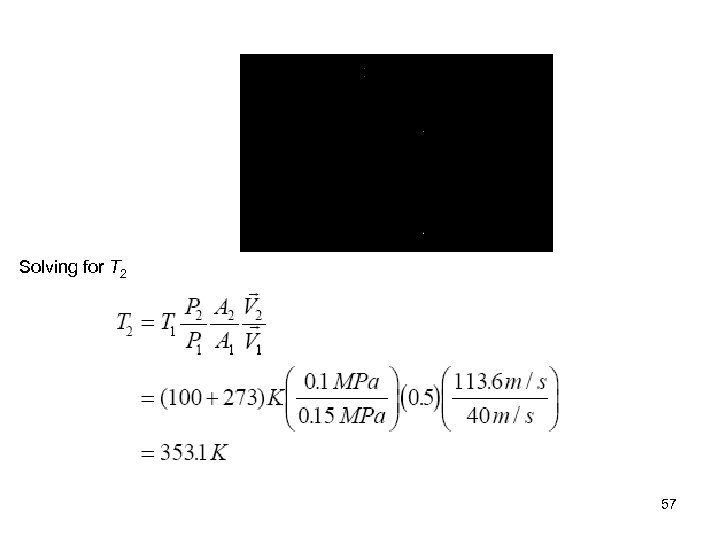Solving for T 2 57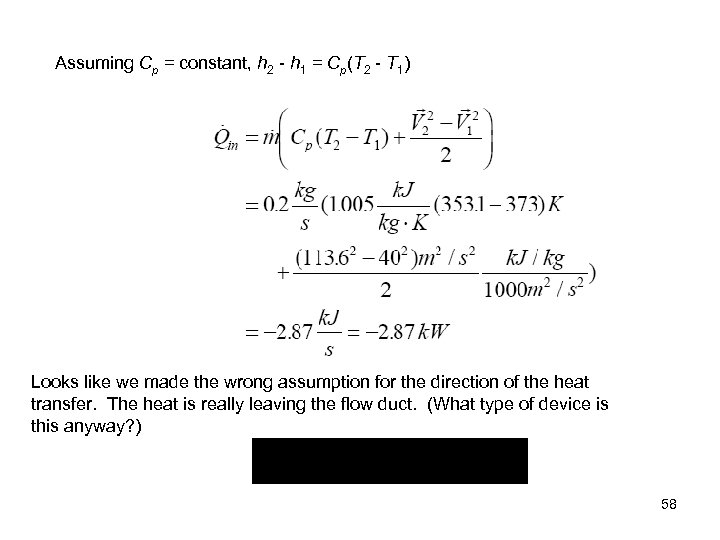Assuming Cp = constant, h 2 - h 1 = Cp(T 2 - T 1) Looks like we made the wrong assumption for the direction of the heat transfer. The heat is really leaving the flow duct. (What type of device is this anyway? ) 58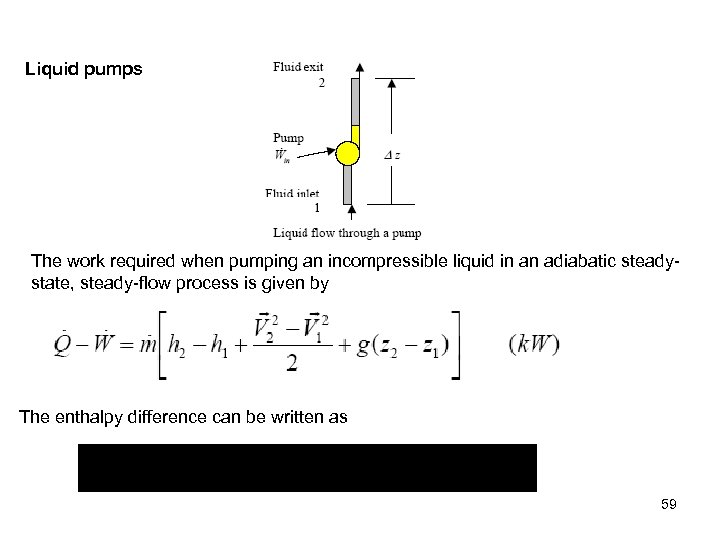Liquid pumps The work required when pumping an incompressible liquid in an adiabatic steadystate, steady-flow process is given by The enthalpy difference can be written as 59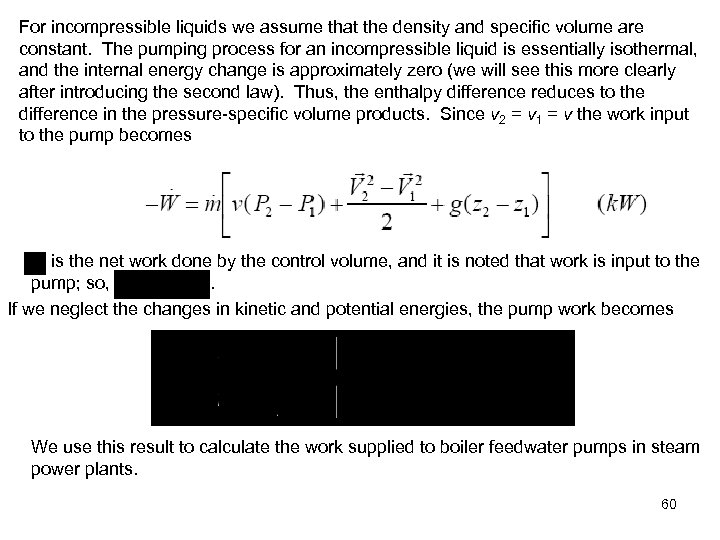For incompressible liquids we assume that the density and specific volume are constant. The pumping process for an incompressible liquid is essentially isothermal, and the internal energy change is approximately zero (we will see this more clearly after introducing the second law). Thus, the enthalpy difference reduces to the difference in the pressure-specific volume products. Since v 2 = v 1 = v the work input to the pump becomes is the net work done by the control volume, and it is noted that work is input to the pump; so, . If we neglect the changes in kinetic and potential energies, the pump work becomes We use this result to calculate the work supplied to boiler feedwater pumps in steam power plants. 60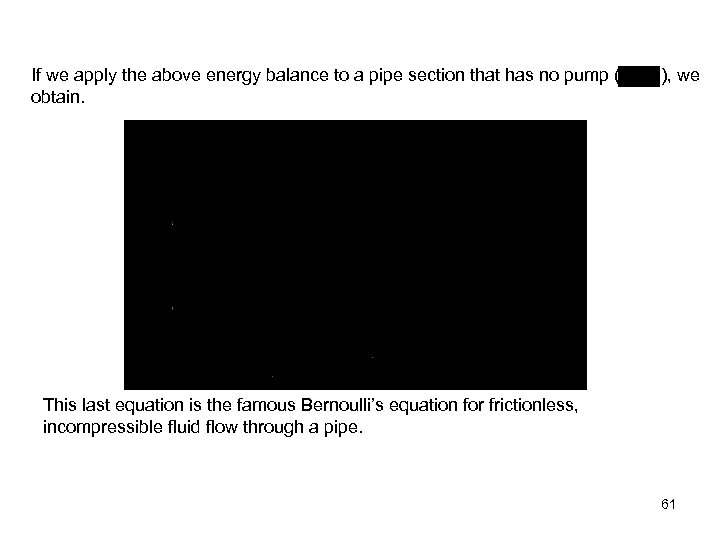If we apply the above energy balance to a pipe section that has no pump ( obtain. ), we This last equation is the famous Bernoulli’s equation for frictionless, incompressible fluid flow through a pipe. 61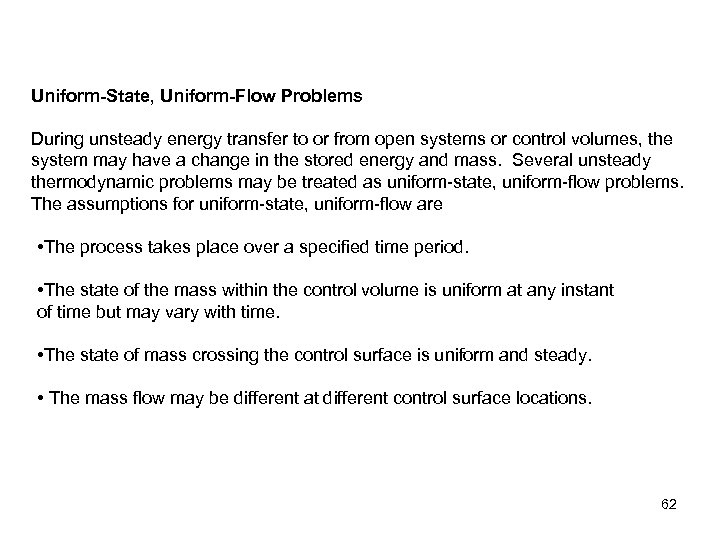Uniform-State, Uniform-Flow Problems During unsteady energy transfer to or from open systems or control volumes, the system may have a change in the stored energy and mass. Several unsteady thermodynamic problems may be treated as uniform-state, uniform-flow problems. The assumptions for uniform-state, uniform-flow are • The process takes place over a specified time period. • The state of the mass within the control volume is uniform at any instant of time but may vary with time. • The state of mass crossing the control surface is uniform and steady. • The mass flow may be different at different control surface locations. 62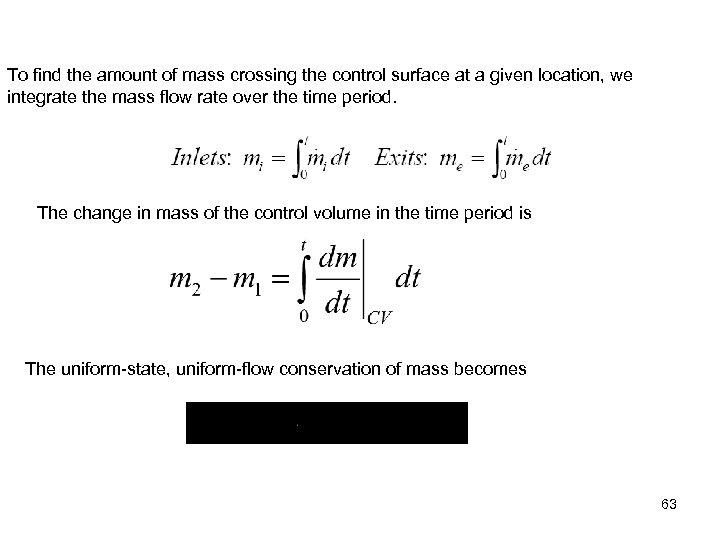To find the amount of mass crossing the control surface at a given location, we integrate the mass flow rate over the time period. The change in mass of the control volume in the time period is The uniform-state, uniform-flow conservation of mass becomes 63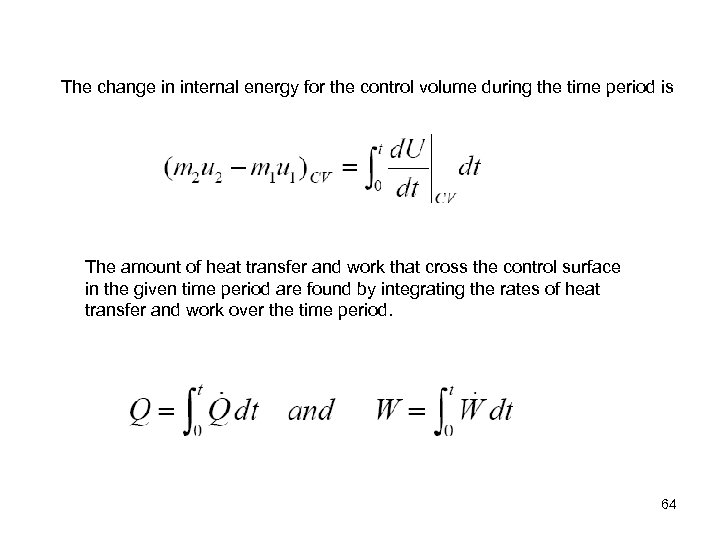The change in internal energy for the control volume during the time period is The amount of heat transfer and work that cross the control surface in the given time period are found by integrating the rates of heat transfer and work over the time period. 64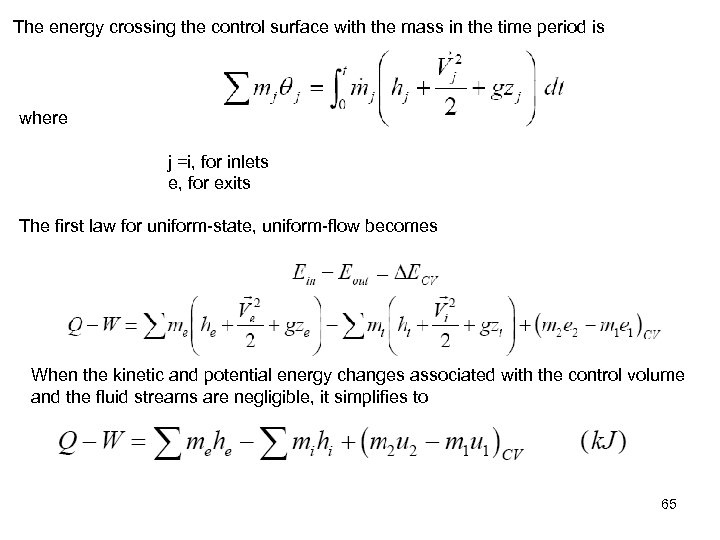The energy crossing the control surface with the mass in the time period is where j =i, for inlets e, for exits The first law for uniform-state, uniform-flow becomes When the kinetic and potential energy changes associated with the control volume and the fluid streams are negligible, it simplifies to 65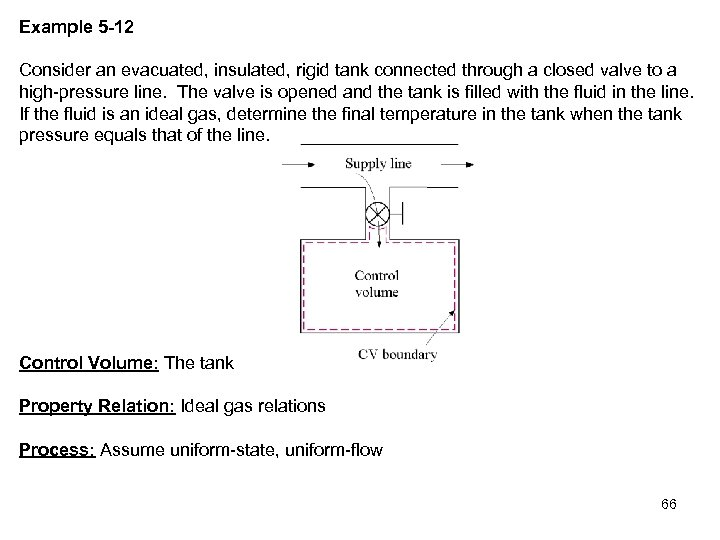Example 5 -12 Consider an evacuated, insulated, rigid tank connected through a closed valve to a high-pressure line. The valve is opened and the tank is filled with the fluid in the line. If the fluid is an ideal gas, determine the final temperature in the tank when the tank pressure equals that of the line. Control Volume: The tank Property Relation: Ideal gas relations Process: Assume uniform-state, uniform-flow 66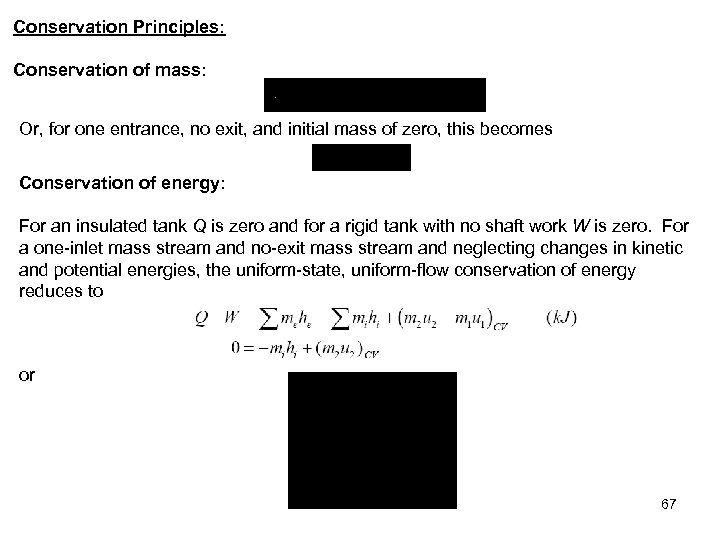Conservation Principles: Conservation of mass: Or, for one entrance, no exit, and initial mass of zero, this becomes Conservation of energy: For an insulated tank Q is zero and for a rigid tank with no shaft work W is zero. For a one-inlet mass stream and no-exit mass stream and neglecting changes in kinetic and potential energies, the uniform-state, uniform-flow conservation of energy reduces to or 67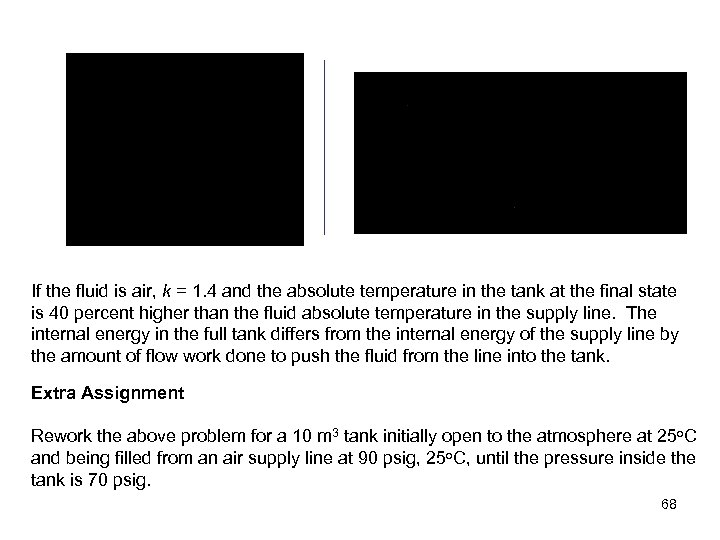If the fluid is air, k = 1. 4 and the absolute temperature in the tank at the final state is 40 percent higher than the fluid absolute temperature in the supply line. The internal energy in the full tank differs from the internal energy of the supply line by the amount of flow work done to push the fluid from the line into the tank. Extra Assignment Rework the above problem for a 10 m 3 tank initially open to the atmosphere at 25 o. C and being filled from an air supply line at 90 psig, 25 o. C, until the pressure inside the tank is 70 psig. 68